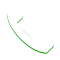0

# How long will a 12v lead acid battery last when connected on a 500 watts inverter subjected to a 65 watts load?

1. There is no way to answer this question unless the battery capacity and type is known. How many amp-hours is the battery? Is it designed for deep cycle use or is it a starting battery? 2. The maximum load capacity of the inverter is not particularly relevant. The efficiency is. If you assume 90% efficiency with a 65-watt load then the inverter is drawing about 72 watts. 72 w / 12 v = 6 amps. If you had a 60AH battery a simple calculation is it would last 10 hours. But there are other factors. For one thing, the battery doesn’t stay at 12 volts as it discharges. As the battery voltage drops, the inverter is going to draw more current to try to keep the output voltage constant.For COD  Orders Call Us